# AP Board 4th Class Maths Solutions 5th Lesson Multiplication

Andhra Pradesh AP Board 4th Class Maths Solutions 5th Lesson Multiplication Textbook Exercise Questions and Answers.

## AP State Syllabus 4th Class Maths Solutions Chapter 5 Multiplication

I.

Nikhila, along with her family members went to an exhibition.They were two children and three adult in all. The entrance – fee for adult is ₹ 120 and for child is ₹ 65. Nikhila’s fathergave ₹ 500 to buy tickets.
How much does he need to pay for the ticket;
“Can you tell how much it is” ?
Rajani worked out it as follows ………….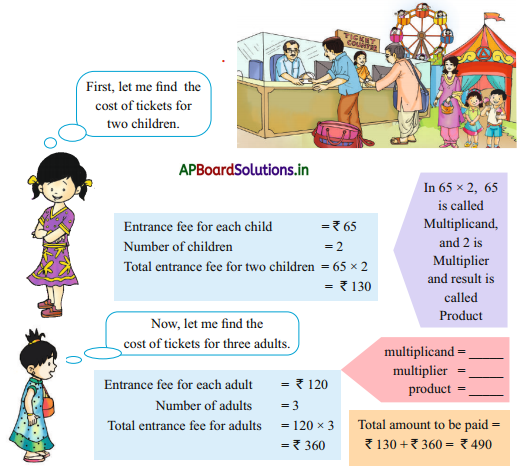Solution:
Multiplicand =         120
Multiplier =          3
Product =         360

II.

If three children and four adults of your family went to the exhibition, then how much do you need to pay ?
Cost of ticket for each child = ____________
Number of children went to the exhibition = ____________
Total cost of tickets for children = ____________
Cost of ticket for each adult = ____________
Number of adults went to the exhibition = ____________
Total cost of tickets for adults = ____________
Total cost of tickets for children and adults = ____________
Solution:
Cost of ticket for each child =        ₹ 65

Number of children went to the exhibition =            3Total cost of tickets for children
= 3 × 65
= 185

Cost of ticket for each adult =          ₹ 120

Number of adults went to the exhibition =            4

Total cost of tickets for adults
= 4 × 120
= ₹ 480

Total cost of tickets for children and adults
= 1 × 5 + 480
= ₹ 665

Textbook Page No. 59

Do this

Question 1.
In the multiplication fact 124 × 2 = 248, write multiplicand, multiplier and the product
Solution:
Multiplicand = 124
Multiplier = 2
Product = 248

2. Find the products.

a) 234 × 2
Solution:
486

b) 232 × 3
Solution:
696c) 212 × 4
Solution:
848

d) 440 × 2
Solution:
880

Let us observe:
Observe the following multiplications and fill in the blanks.
1) 1 × 200 =           200
2) 2 × 200 =           400
3) 3 × 200 =           600
4) 4 × 200 =           800
5) 5 × 200 =           1000
6) 6 × 200 =           1200
7) 7 × 200 =           1400
8) 8 × 200 =           1600
9) 9 × 200 =           1800
10) 10 × 200 =           2000

1) 1 × 300 =           300
2) 2 × 300 =           600
3) 3 × 300 =           900
4) 4 × 300 =           1200
5) 5 × 300 =           1500
6) 6 × 300 =          1800
7) 7 × 300 =           2100
8) 8 × 300 =           2400
9) 9 × 300 =           2700
10) 10 × 300 =           30001) 1 × 400 =           400
2) 2 × 400 =           800
3) 3 × 400 =          1200
4) 4 × 400 =           1600
5) 5 × 400 =           2000
6) 6 × 400 =           2400
7) 7 × 400 =           2800
8) 8 × 400 =           3200
9) 9 × 400 =           3600
10) 10 × 400 =           4000

Textbook Page No. 60

Do this

Write the following products.

1) 11 × 200 =          2200         ; 200 × 1 =          2200
2) 13 × 200 =          2600           ; 200 × 13 =          2600
3) 12 × 300 =          3600           ; 300 × 12 =          3600
4) 14 × 300 =          4200           ; 300 × 14 =          4200
5) 12 × 400 =          4800           ; 400 × 12 =          4800
6) 14 × 400 =          5600           ; 400 × 14 =          5600
7) 4 × 500 =          2000           ; 500 × 4 =          2000
8) 6 × 500 =          3000           ; 500 × 6 =          3000

Textbook Page No. 62

Do this

1. Find the products in the above methods and observe the answers.

a) 164 × 2
Solution:
Given: 164 × 2
Method – I:
164 × 2 = (100 + 60 + 4) × 2
= 100 × 2 + 60 × 2 + 4 × 2
= 200 + 120 + 8
= 328Method – II: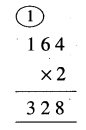Method – III: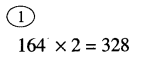In three methods product is same.

b) 246 × 3
Solution:
Given: 246 × 3
Method – I:
246 × 3 = (200 + 40 + 6) × 3
= (200 × 3 + 40 × 3 + 6× 3)
= 600 + 120 + 8
= 600 + 138
= 738

Method – II: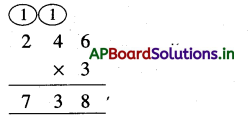Method – III: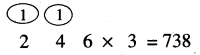In three methods product is same.c) 209 × 4
Solution:
Given: 209 × 4
Method – I:
209 × 4 = (200 + 0 + 9) × 4
= (200 × 4 + 0 × 4 + 9 × 4)
= 800 + 00 + 36
= 836

Method – II: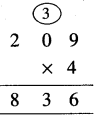Method – III: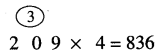In three methods product is same.

2. Verify the following and correct them wherever necessary.

a) 264 × 2
Solution:
Given 264 × 2 = 4,128.
It is wrong.
Correct multiplication = 264 × 2 = 528

b) 342 × 3
Solution:
Given 342 × 3 = 1026 is correct.c) 253 × 4
Solution:
Given 253 × 4 = 82012 is wrong.
Correct multiplication: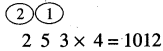is correct.

Textbook Page No. 62

Try this

Question 1.
In Chammachintha school, there are 126 pupils. The teacher asked them to plant saplings in the nearby village. If each student planted 4 saplings, how many saplings did they plant ?
Solution:
Number of students in the school = 126
Number of saplings planted by each student = 4
Total saplings planted by the students
= 126 × 4
= 504

Question 2.
If a box contains 164 mangoes, how many mangoes will be there in 5 such boxes ?
Solution:
Number of mangoes in a box = 164
Number of mangoes such boxes = 5
Total mangoes in 5 boxes
= 164 × 5
= 820 mangoesQuestion 3.
Ramayya went to a agriculture work for 2 days. If his wage was ₹ 425 per day, what was the total wage for 2 days ?
Solution:
Daily wage per day = 425
Number of working days = 2
Total wage for 2 days = 2 × 425
= ₹ 850

Textbook Page No. 63

Do this

Do the following by above method.

a) 114 × 3 = ___________.
Solution:
114 × 3
= (1 hundred + 1 tens + 4 ones) × 3
= 3 hundred + 3 tens + 2 ones
= 3 hundred + (3 tens + 1 ten) + 2 ones
= 300 + 40 + 2

b) 314 × 4 = ____________
Solution:
314 × 4
= (3 hundreds + 1 ten + 4 ones) × 4
= 12 hundreds + 4 tens + 1 ten + 16 ones
= 12 hundreds + 4 tens + 1 ten + 6 ones
= 1200 + 40 + 10 + 6
= 1200 + 50 + 6
= 1256c) 213 × 5 = ___________
Solution:
214 × 5
= (2 hundreds + 1 ten + 3 ones) × 5
= 10 hundreds + 5 tens + 15 ones
= 10 hundreds + 5 tens + 1 ten + 5 ones
= 10 hundreds + 6 tens + 5 ones
= 1000 + 60 + 5 = 1065

d) 134 × 6 = ___________
Solution:
134 × 6
= (1 hundred + 3 tens + 4 ones) × 6
= 6 hundreds + 18 tens + 24 ones
= 6 hundreds +10 tens + 8 tens + 2 tens + 4 ones
= 600+ 100 + 80 + 20 + 4
= 700 + 100 + 4
= 804

e) 243 × 5 = ____________
Solution:
243 × 5
= (2 hundreds + 4 tens + 3 ones) × 5
= 10 hundreds + 20 tens + 15 ones
= 1000 + 20 tens + 1 ten + 5 ones
= 1000 + 200+ 1 ten+ 5
= 1000 + 210 + 5
= 1215f) 126 × 7 = __________
Solution:
126 × 7
= = (1 hundred + 2 tens + 6 ones) × 7
= 7 hundreds + 14 tens +42 ones
= 700 + 140 + 40 + 2
= 882

Textbook Page No. 65

Do this

Do the following multiplications.

a) 342 × 15
Solution: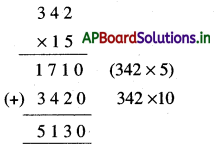b) 423 × 21
Solution: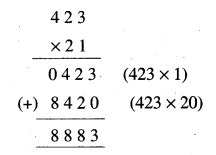c) 233 × 26
Solution: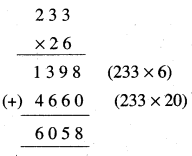Textbook Page No. 65

Try this

Question 1.
how many trees are there in the garden, if there are 124 rows and each row contains 65 trees?
Solution:
Number of rows in a garden = 124
Number of trees in each row = 65
Number of trees totally in the garden =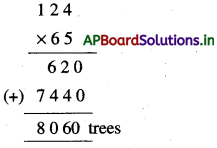Question 2.
The owner of a dairy farm sold 496 milk packets in 15 days. If each packet was sold at ₹ 25, how much money did he earn ?
Solution:
Number of milk packets sold = 496
Cost of each packet = 25
Total earned money =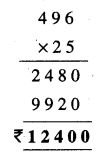Exercise – 5.1

1. Multiply

a) 348 × 27
Solution: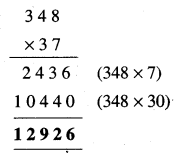b) 456 × 48
Solution: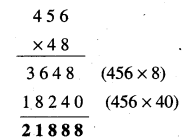c) 654 × 55
Solution: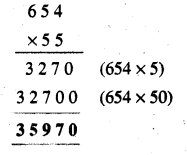d) 708 × 64
Solution: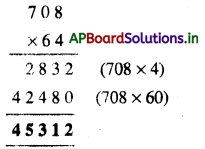Question 2.
The cost of a chair is ₹ 375. What is the cost of 18 such chairs ?
Solution:
Cost of a chair is = ₹ 375
Cost of such 18 chairs is =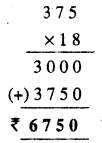Question 3.
Raju delivers 157 newspapers each morning. How many newspapers does he deliver in 31 days ?
Solution:
Number of newspapers delivers each mornings = 157
Number of days = 31
∴ Total newspapers delivered in 31 days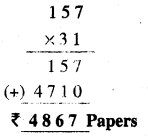Question 4.
42 boys in a class planned a picnic. If each boy contributes ₹ 168, how much money was collected ?
Solution: N
umber of boys in the class = 42
Contribution of each boys = ₹ 168
∴ Collected money totally =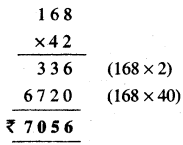Question 5.
The bus fare from Vizag to Vijayawada is ₹ 650. If 28 passengers travelled from Vizag to Vijayawada, how much money have they paid ?
Solution:
The bus fare from Vizag to Vijayawada = ₹ 650
Number of passengers in the bus = 28
Total money paid by the passengers =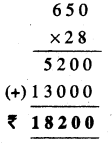Question 6.
Raju buys 65 crates of mangoes at ₹ 285 per crate. How much money does he pay ?
Solution:
Number of crates purchased = 65
Cost of each crate = ₹ 285
Totally paid amount =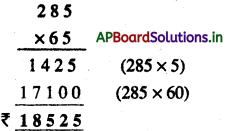Question 7.
There are 576 buttons in a packet. Find the number of buttons in 2 such packets.
Solution:
Number of buttons in a packet = 576
Number of buttons in packets = 82
Total buttons in the packets =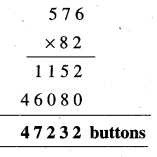write down here :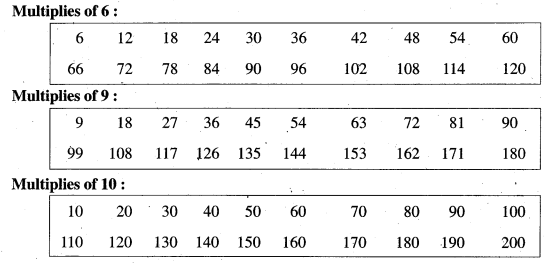Exercise – 5.2

1. Find the first 5 multiplies of each of the following.

a) 3
Solution:
3 × 1 = 3
3 × 2 = 6
3 × 3 = 9
3 × 4 = 12
3 × 5 =15

b) 7
Solution:
7 × 1 = 7
7 × 2 =14
7 × 3 = 21
7 × 4 = 28
7 × 5 = 35c) 8
Solution:
8 × 1 =8
8 × 2 = 16
8 × 3 = 24
8 × 4 = 32
8 × 5 = 40

Question 2.
Write the multiples of 8 below 100.
Solution:
Multiples of 8, below 100 is 8, 16, 24, 32, 40, 48, 56, 64, 72, 80, 88, 96.

Question 3.
Read each of the following statements. If it is true write ‘T’, otherwise ‘F’ in the bracket.
a) 12 is a multiple of 3
Solution:
T

b) 57 is a multiple of 8
Solution:
F

c) 30 is a multiple of 5
Solution:
Td) 47 is a multiple of 6
Solution:
F

e) 52 is a multiple of 7
Solution:
F

Question 4.
Circle the multiples of 3 in the following numbers.
2, 5, 6, 9, 10, 14, 20, 21, 27, 32, 37, 36, 48.
Solution: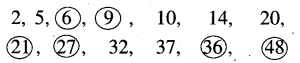Question 5.
Circle the numbers which are not multiples of 4 in the following numbers.
2, 4, 11, 8, 20, 21, 27, 28, 30, 32, 37, 40, 45, 57.
Solution: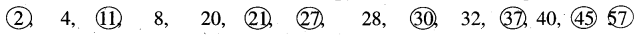Question 6.
Circle the multiples of 5 in the following numbers.
2, 4, 14, 20, 21, 27, 35, 55, 25, 68, 65, 22, 39.
Solution: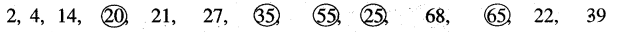Question 7.
Write the numbers which are not multiples of 8 in the following numbers.
20, 24, 45, 32, 35, 26, 90, 8, 7, 10.
Solution: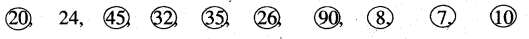Multiple Choice Questions

Question 1.
Choose in 120 × 65, multiplicand
A) 120
B) 65
C) 780
D) None
A) 120

Question 2.
Choose in 145 × 3, multiplier
A) 145
B) 3
C) 435
D) 534
B) 3Question 3.
If two numbers are multiplied in any order the product is always
A) Negative
B) Positive
C) Same
D) None
C) Same

Question 4.
The product value of 16 hundreds + 9 tens + 2 ones is equal to
A) 423 × 5
B) 4 × 423
C) 3 × 423
D) 423 × 6
B) 4 × 423

Question 5.
A weaver’s family produced 23 sarees. They sold each saree at ₹ 385. How much money they earn ?
A) 5588
B) 5508
C) 8855
D) 8805
C) 8855Question 6.
Product of 348 × 37 is
A) 12726
B) 12826
C) 12876
D)12626
C) 12876

Question 7.
Sohan done the product 253 × 4 = 82012. Is Sohan correct ?
A) Yes
B) No
C) I don’t know
D) Both A and B
B) No

Question 8.
Is 45 is a multiple of 8
A) Yes
B) No
C) I don’t know
D) Both A and B
B) NoQuestion 9.
Raju said that “13 × 100 = 100 × 13 is same”. Is Raju correct ?
A) Yes
B) No
C) I don’t know
D) Both A and B